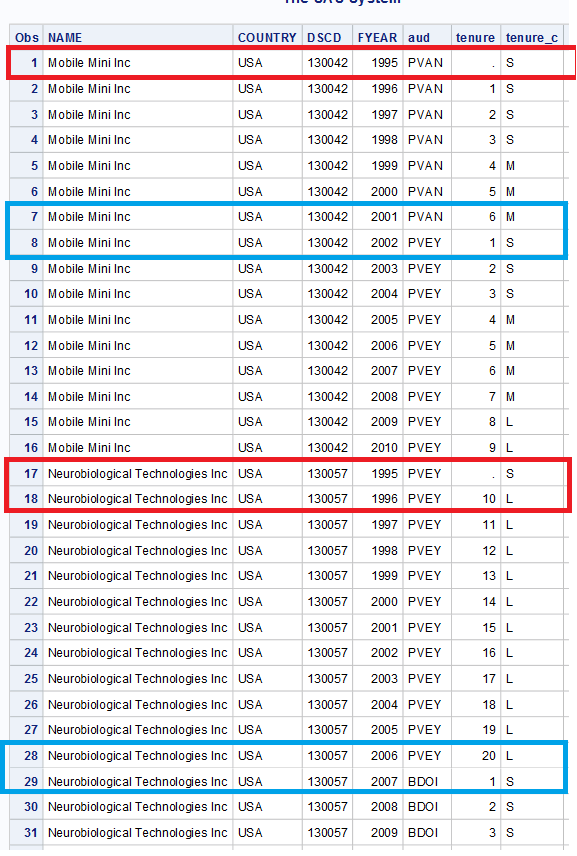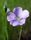## Counting consecutive variable values

Hello Fellow SAS Users,

I have been working on something for a few weeks and just ran into a problem with my code. Esentially, I need SAS to count the number of consecutive variable values (including restarting values) separately for each participant.

(I am not going to include my current SAS code, because I am sure it is very inefficient and there is a much better way to achieve this.)

I have two problems, but first take a look at an example of the output:

NAME = Participant

DSCD = Identifier

FYEAR = Year of observation

AUD = the variable for which I need to count the number of repititions

TENURE = the count of the repition of AUD, as of the current FYEARMy two problems are summarized as follows (based no the two red boxes😞

1. First, the very first observation for each participant should be where TENURE=1. My code is showing as TENURE=. and then starting TENURE=1 in the second observation.

2. The more important problem is what happens in the second participant. The same problem as #1 above persists. But then in FYEAR=1996 for this participant, it is continuing off of the previous count string.

Yet, interestingly, for both participants shown here (look at the BLUE boxes, FYEAR=2002 for the first and FYEAR=2007 for the second), when there is a change in the AUD variable, neither of the two preceding problems are present. The first year of the new AUD variable is = 1, and it restarts the count rather than pulling from the previous count.

I would appreciate any help with this. Again, I'm sorry for not including my current SAS code but I am very confident that it is wasteful and inefficient.

4 REPLIES 4

## Re: HELP NEEDED ASAP: Counting consecutive variable values

Update: I am trying to create a simple DO loop and this is also giving me problems. Here's the code I'm working with for that:

``````*COMPUTE TENURE (IN YEARS) AND CLASSIFY AS S/M/L*;
data tenure; set merged;
aud=aud2;
dscd1 = lag(dscd);
aud1 = lag(aud);

if dscd ne dscd1 then tenure=1;
else if dscd=dscd1 then do;
tenure1=lag(tenure);
if aud=aud1 then tenure = tenure1+1;
if aud ne aud1 then tenure = 1;
end;

if tenure <=3 then tenure_c='S';
else if 7 >= tenure > 3 then tenure_c='M';
else if tenure > 7 then tenure_c='L';
else if tenure='.' then tenure_c='.';

run;``````

## Re: HELP NEEDED ASAP: Counting consecutive variable valuesLinusH
Tourmaline | Level 20

## Re: HELP NEEDED ASAP: Counting consecutive variable values

First - nothing on this community can be ASAP. All entries are voluntary.

Second - if you figured out how to do it - share! Some om your peers can benefit from this. And it's nice to couple a solution to your question.

Data never sleeps

## Re: HELP NEEDED ASAP: Counting consecutive variable values

I realize this post is 3 years-old (and the original poster had already resolved the issue him/herself), but I have found myself in a similar situation, stumbling across a SAS community question that perfectly replicates the question I'm trying to sort out, with no final resolution. Just in case others are struggling to find syntax to create a count variable, here is some code to help:

First, sort data by your grouping variable and the variable you want counted. ID = grouping variable; Date = ordering variable

``````proc sort data=one;
by ID Date;
run;``````

Next, create a count variable in a new data set. In this case, each first ID starts at a count of 1, and continues to count up until the next ID.

``````data two;
set one;
*Create count variable.;
Count + 1;
by ID;
if first.ID then Count = 1;
run;``````

If you wanted to create a running count within more than one category, for example, number of observations within each participant and date, you can simply add the additional variable to your count syntax after the "by" statement (e.g., "by ID Date").

If you need something slightly more complicated, for example, counting only certain types of observations, some additional syntax is listed below.

``````data two;
set one;
*Create drinking observation count.;
if Drinking=1 then Count + 1;
by ID;
if first.ID then Count = 1;
run;``````

Here, I created a count of drinking observations (only when drinking = 1, not drinking = 0) within each participant ID.

Discussion stats
• 4 replies
• 4354 views
• 0 likes
• 3 in conversation